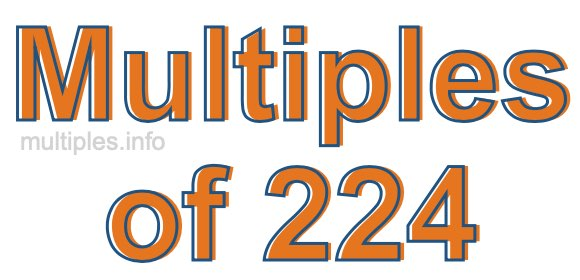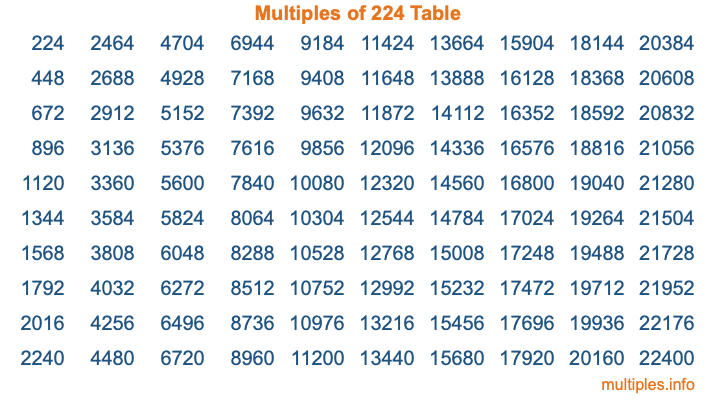Multiples of 224Welcome to the Multiples of 224 page. Here we will first teach you everything you will ever need to know about the multiples of 224, and then give you a study guide summary of everything we taught you to make sure you remember it all. Use this page to look up facts and learn information about the multiples of 224. This page will make you a multiples of two hundred twenty-four expert!

Definition of Multiples of 224
Multiples of 224 are all the numbers that when divided by 224 equal an integer. Each of the multiples of 224 are called a multiple. A multiple of 224 is created by multiplying 224 by an integer.

Therefore, to create a list of multiples of 224, you start with 1 multiplied by 224, then 2 multiplied by 224, then 3 multiplied by 224, and so on for as long as you want. Thus, the list of the first five multiples of 224 is 224, 448, 672, 896, and 1120. To see a larger list of multiples of 224, see the printable image of Multiples of 224 further down on this page. We also have a category where you can choose any nth multiple of 224.

Multiples of 224 Checker
The Multiples of 224 Checker below checks to see if any number of your choice is a multiple of 224. In other words, it checks to see if there is any number (integer) that when multiplied by 224 will equal your number. To do that, we divide your number by 224. If the the quotient is an integer, then your number is a multiple of 224.

Is  a multiple of 224?

Least Common Multiple of 224 and ...
A Least Common Multiple (LCM) is the lowest multiple that two or more numbers have in common. This is also called the smallest common multiple or lowest common multiple and is useful to know when you are adding our subtracting fractions. Enter one or more numbers below (224 is already entered) to find the LCM.

Check out our LCM Calculator if you need more details about the Least Common Multiple or if you need the LCM for different numbers for adding and subtraction fractions.

nth Multiple of 224
As we stated above, 224 is the first multiple of 224, 448 is the second multiple of 224, 672 is the third multiple of 224, and so on. Enter a number below to find the nth multiple of 224.

th multiple of 224

Multiples of 224 vs Factors of 224
224 is a multiple of 224 and a factor of 224, but that is where the similarities end. All postive multiples of 224 are 224 or greater than 224. All positive factors of 224 are 224 or less than 224.

Below is the beginning list of multiples of 224 and the factors of 224 so you can compare:

Multiples of 224: 224, 448, 672, 896, 1120, etc.

Factors of 224: 1, 2, 4, 7, 8, 14, 16, 28, 32, 56, 112, 224

As you can see, the multiples of 224 are all the numbers that you can divide by 224 to get a whole number. The factors of 224, on the other hand, are all the whole numbers that you can multiply by another whole number to get 224.

It's also interesting to note that if a number (x) is a factor of 224, then 224 will also be a multiple of that number (x).

Multiples of 224 vs Divisors of 224
The divisors of 224 are all the integers that 224 can be divided by evenly. Below is a list of the divisors of 224.

Divisors of 224: 1, 2, 4, 7, 8, 14, 16, 28, 32, 56, 112, 224

The interesting thing to note here is that if you take any multiple of 224 and divide it by a divisor of 224, you will see that the quotient is an integer.

Multiples of 224 Table
Below is an image of the first 100 multiples of 224 in a table. The table is in chronological order, column by column. The first column has the first ten multiples of 224, the second column has the next ten multiples of 224, and so on.The Multiples of 224 Table is also referred to as the 224 Times Table or Times Table of 224. You are welcome to print out our table for your studies.

Negative Multiples of 224
Although not often discussed or needed in math, it is worth mentioning that you can make a list of negative multiples of 224 by multiplying 224 by -1, then by -2, then by -3, and so on, to get the following list of negative multiples of 224:

-224, -448, -672, -896, -1120, etc.

Multiples of 224 Summary
Below is a summary of important Multiples of 224 facts that we have discussed on this page. To retain the knowledge on this page, we recommend that you read through the summary and explain to yourself or a study partner why they hold true.

There are an infinite number of multiples of 224.

A multiple of 224 divided by 224 will equal a whole number.

224 divided by a factor of 224 equals a divisor of 224.

The nth multiple of 224 is n times 224.

The largest factor of 224 is equal to the first positive multiple of 224.

224 is a multiple of every factor of 224.

224 is a multiple of 224.

A multiple of 224 divided by a divisor of 224 equals an integer.

224 divided by a divisor of 224 equals a factor of 224.

Any integer times 224 will equal a multiple of 224.

Multiples of a Number
Here you can get the multiples of another number, all with the same attention to detail as we did for multiples of 224 on this page.

Multiples of
Multiples of 225
Did you find our page about multiples of two hundred twenty-four educational? Do you want more knowledge? Check out the multiples of the next number on our list!